# Problems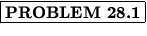If an electron makes a transition from the n = 4 to the n = 1 Bohr orbital in a hydrogen atom, determine the wavelength of the light emitted and the recoil speed of the atom.

Solution:
To determine the wavelength, we use= R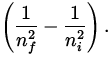With R = 1.097 x 107 m, ni = 4 , and nf = 1 , we find= 97.2 nm. This photon carries away momentum given by

p =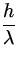=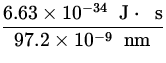= 6.62 x 10- 27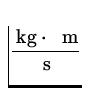,

which by momentum conservation must be the recoil momentum of the atom. Using the mass of the hydrogen atom as 1.67 x 10- 27 kg, we find it's speed must be

v =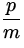=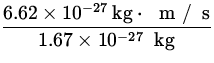= 4.1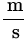.

Thus, the wavelength of the emitted photon is 97.2 nm, while the recoil speed of the hydrogen atom is 4.1 m/s.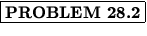A photon incident on a hydrogen atom causes the electron to make a transition from the n = 1 orbital to the n = 3 orbital. What is the wavelength of the photon, and what are the possible wavelengths of the emitted radiation when the electron returns to the n = 1 state?

Solution:
We assume the photon gives up all of its energy to the electron. In making a transition from n = 1 to n = 3 , the electron gains an energy

E = - 13.6 eV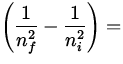- 13.6 eV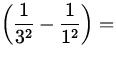12.1 eV ,

which by energy conservation must be equal to the energy of the incident photon. The associated wavelength is then found using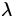==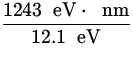= 102.8 nm .

When the electron in the excited n = 3 state falls back to the ground state with n = 1 , it can do so by 2 distinct paths: 3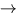1 and 321 . This would correspond to 3 distinct wavelengths of the emission spectra.

Thus, the wavelength of the incident photon is 102.8 nm, and there would be 3 possible wavelengths present in the emitted radiation when the electron returned to the n = 1 state.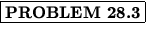An electron is in the first Bohr orbital of the hydrogen atom. Determine the speed of the electron, the time it takes to complete one complete revolution, and the current in Ampères corresponding to this moving charge.

Solution:
The energy of the electron is given by

E = -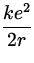= -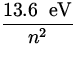,

which in the first Bohr orbital with n = 1 corresponds to an energy of - 13.6 eV. Using the relations=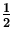mv 2,

we find this corresponds to a kinetic energy ofmv 2 = - E = 13.6 eV x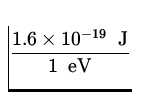= 2.2 x 10- 18 J .

From this we obtain the speed as

v ==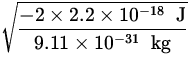= 2.2 x 106.

To obtain the time taken to complete one revolution we divide the distance travelled ( = 2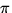a0 = 2x 0.053 nm) by the speed:

T =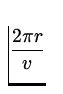== 1.52 x 10- 16 s .

This corresponds to a current of

I =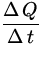=== 1.1 x 10- 3 A .

Thus, the speed of the electron in 2.2 x 106 m/s, it takes 1.52 x 10- 16 s to complete one revolution, and the associated current is 1.1 x 10- 3 A.Consider a string fixed at both ends which vibrates in a standing wave pattern, as in Fig. 28.3.Suppose such a wave pattern describes a free particle of mass m confined to a box of length L . Find the allowable energy levels of such a particle.

Solution:
In order for a standing wave to exist between the ends, we must have a half integral or else an integral number of wavelengths present:

n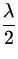= L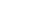n = 1,2,3,...

Using the de Broglie hypothesis, we can relate this wavelength to the momentum of the particle as=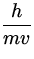=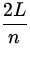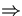v =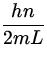.

From this, we find the kinetic energy of the particle to be

En =mv 2 =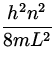.

Thus, the energy of the particle is quantized as E = E0n 2, where E0 = h 2/8mL 2.Write out the possible electronic energy states for an electron in the n = 1 and 2 energy levels.

Solution:
For a given value of n , the orbital quantum number can assume values l = 0,1,2,...,n - 1 , the orbital magnetic quantum number can be ml = - l, - l + 1,...,0,...,l - 1,l , and the spin quantum number can be ms =.

For n = 1 we have only l = 0 , for which ml = 0 is the only allowed value. Thus, we find

 n l ml ms 1 -1 +For n = 2 , l can assume values 0 (with ml = 0 ) or 1 (for which ml = - 1,0,1 ). We then have

 n l ml ms 2 -2 +2 1 - 1 -2 1 - 1 +2 1 -2 1 +2 1 + 1 -2 1 + 1 +Note that at this point we cannot say which of the various states for a given value of n have lower energy.